Let's do another one where we get a bit of a head start: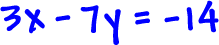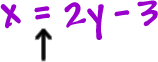Notice that one equation is solved for
x...

Let's stick that x blob into the other equation in place of x:This gives us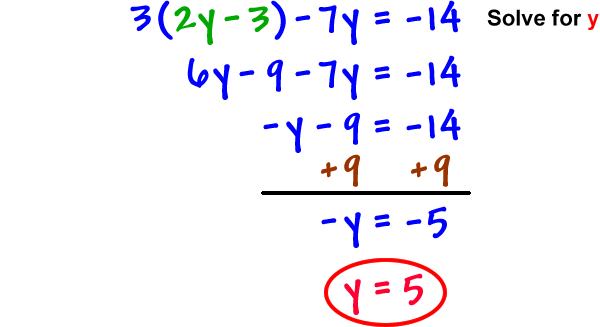OK, we've got y...  Now, we need x...  See the circled blob above?

Stick it in there!  ( That's why I circled it! )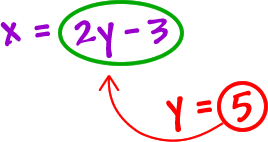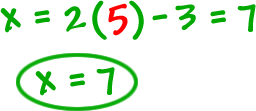Is our answer (7, 5) ?  Let's check...
and you have to check it in BOTH equations!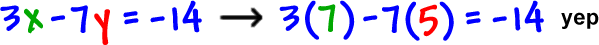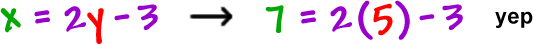So, the answer is (7, 5).

TRY IT:

Solve by substitution: